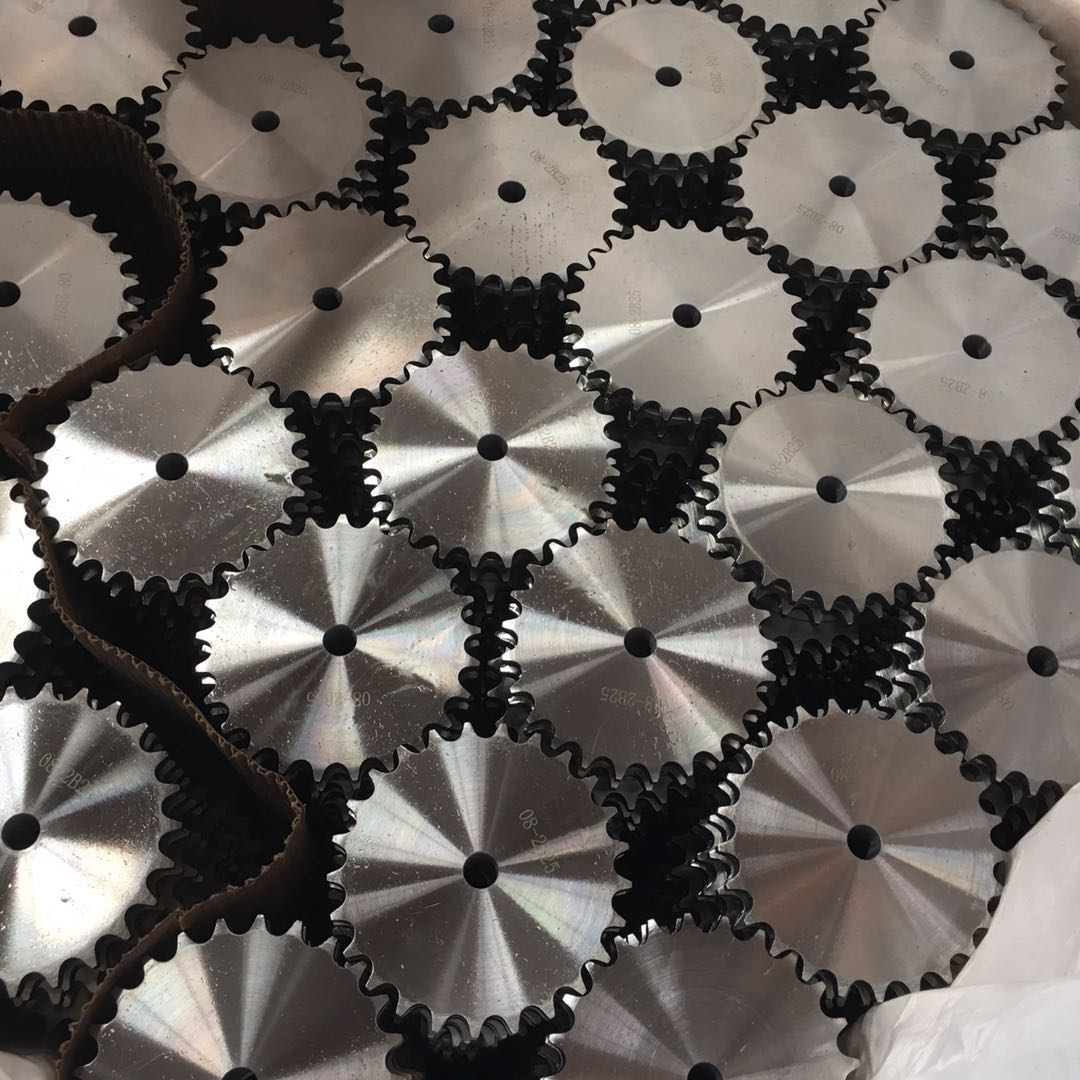Introduction Of Sprocket

# Introduction Of Sprocket

Summary

Introduction of sprocket

Introduction Of Sprocket

The number of teeth is the total number of teeth (protrusions) around the entire circumference of the sprocket. For sprockets with a small number of teeth, it is easy to simply calculate the number of teeth. However, for sprockets with a large number of teeth, trying to calculate the number of teeth may be impractical or inaccurate.
The pitch circle diameter (PD) is an imaginary circle that is tracked by the center of the chain pin when the sprocket is engaged with the chain. The ratio of the pitch circle diameters between the sprockets can be used to calculate the gear ratio, but the more common and simpler is the gear ratio for this calculation.
Pitch represents the amount of pitch circle diameter in inches per tooth. A gear with a larger pitch will have larger teeth. Common pitches are 0.25", called #25, and 0.375". (#35).
The outer diameter (OD) will always be larger than the pitch circle diameter but smaller than the chain gap diameter. The outer diameter does not consider the additional diameter of the chain, so it should not be used to check assembly interference.
The chain gap diameter is the outer diameter of the sprocket that the chain surrounds. The chain gap diameter is always greater than the pitch circle diameter and outer diameter. When placing the sprocket very close to other structures, use the chain gap diameter when checking for interference.